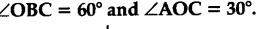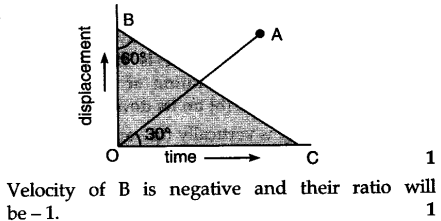# The displacement-time graph of two bodies P and Q are represented by OA and BC respectively. What is the ratio of velocities P and Q?

The displacement-time graph of two bodies P and Q are represented by OA and BC respectively. What is the ratio of velocities P and Q ?